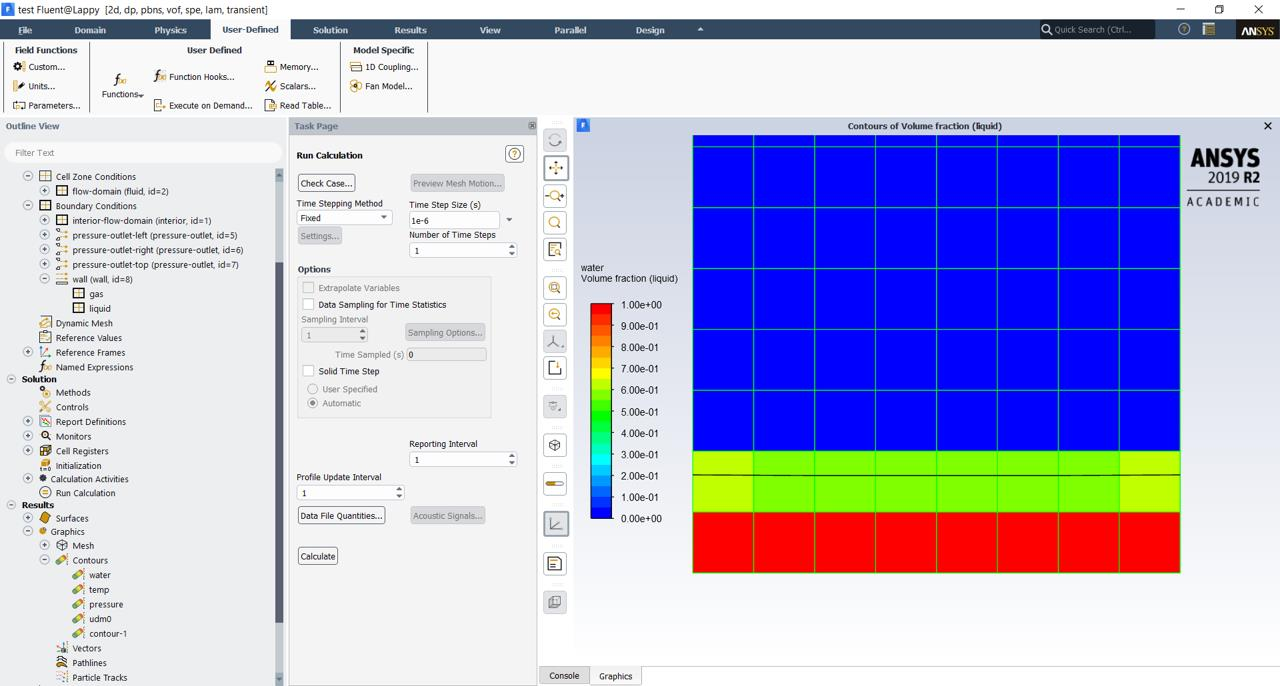## Fluids

•vinitraj3
Subscriber

To all the experts,

I am trying to understand the concept of interfacial area density for a multiphase problem in which water is evaporating into its vapor. Consider a planar surface. Now I i assume that in a cell (2d) of size 0.0125m the volume fraction is 0.4, the UDF returns me rightly the volume of the cell to 0.00015625. Now I am expecting that the interface area should be returned as 0.0125, since the interface should be planar.

The fluent UDF manual shows gives Interfacial area density as magnitude of gradient of alpha, i.e NV_MAG(C_VOF_G(c, ppt)). So if I multiply this with volume I should be expecting Interfacial area. or

Interface Area = NV_MAG(C_VOF_G(c, ppt)) * C_VOLUME(c, ppt)

Now here is interesting thing

When I use the Green Gauss Cell Based Method i get area densities as approximately 59 which when multiplied with 0.00015625 gives me interface area as 0.00932.

However, when I use Green Gauss Node Based Method, i get area densities around 40 which multiplied with volume gives me 0.00625 (half of the expected interface area). So should I be multiplying it with 2.

I am attaching the UDF file as well as the test case which I have been using.

Help needed in understanding why both methods give different area and what is the physical significance of interface area density

•vinitraj3
SubscriberWater volume fraction was patched in the bottom two rows and the black horizontal line shows the water volume fraction=0.5 line. I.e the interface cells are one row above the bottom.

The UDF is also pasted here

// The DEFINE_ADJUST macro is used to calculate the interfacial area density

{

#if !RP_HOST // either serial or node

cell_t c;

// Allocate storage to store the gradients

Domain *pDomain = DOMAIN_SUB_DOMAIN(domain, 0);

{

Alloc_Storage_Vars(pDomain, SV_VOF_RG, SV_VOF_G, SV_NULL);

Scalar_Reconstruction(pDomain, SV_VOF, -1, SV_VOF_RG, NULL);

Scalar_Derivatives(pDomain, SV_VOF, -1, SV_VOF_G, SV_VOF_RG, Vof_Deriv_Accumulate);

}

// Loop over all the cells in the domain

{

begin_c_loop(c, t) // Loop over all the cells in the thread

{

if (C_VOF(c, ppt) != 0.0 && C_VOF(c, ppt) != 1.0) // Check for threads which have interface

{

C_UDMI(c, t, 0) = NV_MAG(C_VOF_G(c, ppt)); // Store the magnitude of gradient of VOF

Message("n Cell Index=%i : Volume fraction=%f: Area Density= %fn", c, C_VOF(c, ppt), C_UDMI(c, t, 0));

//NV_V(A,=,C_VOF_G(c, ppt));

//Message("n Cell Index=%i : Gradient in x =%f", c, A);

//Message("n Cell Index=%i : Gradient in y =%f", c, A);

}

}

end_c_loop(c, t)

}

Free_Storage_Vars(pDomain, SV_VOF_RG, SV_VOF_G, SV_NULL); // Free the storage

#endif // if !RP_HOST

}

//This method of calculation has been picked up from CFD online dot com discussion forum

{

#if !RP_HOST // either serial or node

cell_t c;

face_t f;

// Allocate storage to store the gradients

Domain *pDomain = DOMAIN_SUB_DOMAIN(domain, 0);

{

Alloc_Storage_Vars(pDomain, SV_VOF_RG, SV_VOF_G, SV_NULL);

Scalar_Reconstruction(pDomain, SV_VOF, -1, SV_VOF_RG, NULL);

Scalar_Derivatives(pDomain, SV_VOF, -1, SV_VOF_G, SV_VOF_RG, Vof_Deriv_Accumulate);

}

// Loop over all the cells in the domain

{

begin_c_loop(c, t) // Loop over all the cells in the thread

{

if (C_VOF(c, ppt) != 0.0 && C_VOF(c, ppt) != 1.0) // Check for threads which have interface

{

C_UDMI(c, t, 1) = 2.0 * C_VOF(c,ppt)* NV_MAG(C_VOF_G(c, ppt)); // Interface area is calculated using the formula Area= 2.alpha.magnitude(grad(alpha))

C_UDMI(c, t, 2) = 2.0 * NV_MAG(C_VOF_G(c, ppt)) * C_VOLUME(c, ppt); //  Interface area is calculated using the formula Area = 2* volume * alpha.magnitude(grad(alpha)

Message("n Cell Index=%i : Interface Area1=%f: Interface Area2= %f: Volume=%fn", c, C_UDMI(c, t, 1), C_UDMI(c, t, 2), C_VOLUME(c, ppt));

}

}

end_c_loop(c, t)

}

Free_Storage_Vars(pDomain, SV_VOF_RG, SV_VOF_G, SV_NULL); // Free the storage

#endif // if !RP_HOST

}

•DrAmine
Ansys Employee

interfacial area density is defined to be the ratio of the projected surface area of the bubbles or particles in a cell. Another definition states the volume integral of the area density must be equal to the real surface area. So if one integrate interfacial area density along the normal would lead to 1.

1/If using mixture model or Eulerian Model for phase change there is no need to calculate gradient based Inter-facial area as it is already available option

2/That the value is different for different Green-Gauss approaches that is expected as the two approaches are completely different (node and cell based)

3/You case is bit ill-posed as it is very diffused

4/You will need to sum all UDM'is to get the area

5/If you think that this is defect please get in touch with local support related to the region

•vinitraj3
Subscriber

Thanks Amine,

The case I have posed is on coarse mesh as I was still testing out my UDF. Once I am clear with things I will go for better mesh resolution.

1. My case is a VOF model where I am trying to write a UDF for mass transfer across the interface of a droplet sitting on a heated surface. For this I need to know how to calculate the interface area in each cell.

2. The interface area is a physical quantity, so should not the different Green Gauss approaches return same interface area.

•DrAmine
Ansys Employee

Green Gauss Cell Based and Node Based are completely different stencils to calculate gradients.

•vinitraj3
Subscriber

Thanks Amine,

I understand that they are different methodologies to calculate gradient. Then in that case which one should be chosen for calculation of area

Regards

Rajeev

•DrAmine
Ansys Employee

I would rather worry about the phase change rate as global quantity. Node Based GG approach is more accurate.